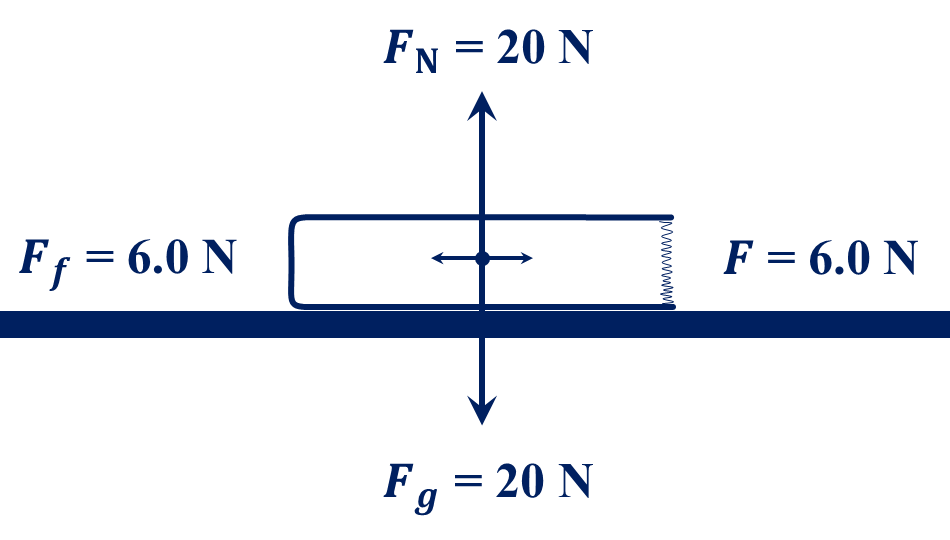﻿ Week 7 Doc O's FHS Physics Assignment Page

Week 7:  17 October to 21 October 2022

17 October 2019:In Class:  Force of Gravity, Normal Force, Weight, and Friction.

Expectations:  Students will solve simple friction problems.

Lab 2b:
Finish the Orienteering Lab (algebraic vectors) Part b.

Expectations:  Students will solve for the resultant of vectors algebraically.

Due:  Pages 1:161-163 (202 – 211).

Homework:  Pages 1:163-164 (212 – 217).

18 October 2022:

In Class:  Relationship between Weight and Friction:

• Ff  =  μ FN
• Ff  = force of friction
μ = coefficient of friction
FN = normal force
• Expectations:  Students will solve friction problems.

Due:  Pages 1:163-164 (212 – 217).

Homework:  Pages 1:164-165 (218 – 227).

19 October 2022:

In Class:  Begin review for the Kinematics Exam (Chapter 1, sections 1 - 6) by begining
the Chap 1 Sect 6 Practice Exam.  Here is the Chap 1 Sect 6 Practice Exam Key to help you.

Expectations:  Students are preparing for the Kinematics Exam.

Due:  Pages 1:164-165 (218 – 227).

Homework:  Finish the Chap 1 Sect 6 Practice Exam.  Here is the